# Search Results (152)

View
Selected filters:
• Functions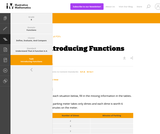Conditions of Use:
No Strings Attached
Rating

This is a task from the Illustrative Mathematics website that is one part of a complete illustration of the standard to which it is aligned. Each task has at least one solution and some commentary that addresses important aspects of the task and its potential use.

Subject:
Functions
Material Type:
Activity/Lab
Provider:
Illustrative Mathematics
Provider Set:
Illustrative Mathematics
Author:
Illustrative Mathematics
02/16/2018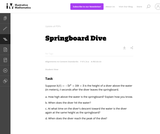Conditions of Use:
No Strings Attached
Rating

The problem presents a context where a quadratic function arises. Careful analysis, including graphing, of the function is closely related to the context. The student will gain valuable experience applying the quadratic formula and the exercise also gives a possible implementation of completing the square.

Subject:
Mathematics
Functions
Material Type:
Activity/Lab
Provider:
Illustrative Mathematics
Provider Set:
Illustrative Mathematics
Author:
Illustrative Mathematics
05/01/2012Conditions of Use:
No Strings Attached
Rating

The problem statement describes a changing algae population as reported by the Maryland Department of Natural Resources. In part (a), students are expected to build an exponential function modeling algae concentration from the description given of the relationship between concentrations in cells/ml and days of rapid growth (F-LE.2).

Subject:
Mathematics
Functions
Material Type:
Activity/Lab
Provider:
Illustrative Mathematics
Provider Set:
Illustrative Mathematics
Author:
Illustrative Mathematics
05/01/2012Conditions of Use:
Remix and Share
Rating

A work in progress, CK-12's Algebra I Second Edition is a clear presentation of algebra for the high school student. Topics include: Equations and Functions, Real Numbers, Equations of Lines, Solving Systems of Equations and Quadratic Equations.

Subject:
Algebra
Functions
Material Type:
Textbook
Provider:
CK-12 Foundation
Provider Set:
CK-12 FlexBook
Author:
Andrew
Annamaria
Anne
Eve
Farbizio
Gloag
Rawley
12/03/2010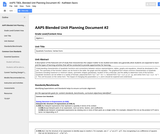Conditions of Use:
Remix and Share
Rating

Understanding characteristics of quadratic functions and connections between various representations, tables, graphs and equations, are developed in this unit. The symmetry of the function values can be found in the table, the graph and the equation. The graphical form shows common characteristics of quadratic functions including maximum or minimum values, symmetric shapes (parabolas), location of the y-intercept, and the ability to determine roots of the function. Quadratic functions can be written in a variety of formats: polynomial form f (x) = ax2 + bx + c, factored form f (x) = a (x -p ) (x - q), and vertex form f (x) = a (x - h) 2 + k. This unit focusses on the vertex form. The impact of changing the parameters a, h, and k will be explored and understood.

Subject:
Mathematics
Functions
Material Type:
Unit of Study
Provider:
Michigan Virtual
Author:
Kathleen Ilaoro
04/17/2017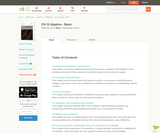Conditions of Use:
Remix and Share
Rating

CK-12 Foundation's Basic Algebra FlexBook is an introduction to the algebraic topics of functions, equations, and graphs for middle-school and high-school students.

Subject:
Algebra
Functions
Material Type:
Textbook
Provider:
CK-12 Foundation
Provider Set:
CK-12 FlexBook
Author:
Farbizio, Annamaria
Gloag, Andrew
Gloag, Anne
Kramer, Melissa
09/21/2010Rating

CK-12 Algebra Explorations is a hands-on series of activities that guides students from Pre-K to Grade 7 through algebraic concepts.

Subject:
Algebra
Functions
Material Type:
Activity/Lab
Lesson Plan
Textbook
Provider:
CK-12 Foundation
Provider Set:
CK-12 FlexBook
Author:
Mary Cavanagh, Carol Findell, Carole Greenes
10/04/2011Conditions of Use:
No Strings Attached
Rating

In this real world problem students solve questions based on the relationship between production costs and price.

Subject:
Mathematics
Functions
Material Type:
Activity/Lab
Provider:
Illustrative Mathematics
Provider Set:
Illustrative Mathematics
Author:
Illustrative Mathematics
05/01/2012Conditions of Use:
No Strings Attached
Rating

This task provides a real world context for interpreting and solving exponential equations. There are two solutions provided for part (a). The first solution demonstrates how to deduce the conclusion by thinking in terms of the functions and their rates of change. The second approach illustrates a rigorous algebraic demonstration that the two populations can never be equal.

Subject:
Mathematics
Functions
Material Type:
Activity/Lab
Provider:
Illustrative Mathematics
Provider Set:
Illustrative Mathematics
Author:
Illustrative Mathematics
05/01/2012Conditions of Use:
No Strings Attached
Rating

This task could be put to good use in an instructional sequence designed to develop knowledge related to students' understanding of linear functions in contexts. Though students could work independently on the task, collaboration with peers is more likely to result in the exploration of a range of interpretations.

Subject:
Mathematics
Functions
Material Type:
Activity/Lab
Provider:
Illustrative Mathematics
Provider Set:
Illustrative Mathematics
Author:
Illustrative Mathematics
05/01/2012Conditions of Use:
No Strings Attached
Rating

This task involves a fairly straightforward decaying exponential. Filling out the table and developing the general formula is complicated only by the need to work with a fraction that requires decisions about rounding and precision.

Subject:
Mathematics
Functions
Material Type:
Activity/Lab
Provider:
Illustrative Mathematics
Provider Set:
Illustrative Mathematics
Author:
Illustrative Mathematics
05/01/2012Conditions of Use:
No Strings Attached
Rating

This task describes two linear functions using two different representations. To draw conclusions about the quantities, students have to find a common way of describing them. We have presented three solutions (1) Finding equations for both functions. (2) Using tables of values. (3) Using graphs.

Subject:
Mathematics
Functions
Material Type:
Activity/Lab
Provider:
Illustrative Mathematics
Provider Set:
Illustrative Mathematics
Author:
Illustrative Mathematics
05/01/2012Conditions of Use:
No Strings Attached
Rating

The purpose of this task is for students to interpret two distance-time graphs in terms of the context of a bicycle race. There are two major mathematical aspects to this: interpreting what a particular point on the graph means in terms of the context, and understanding that the "steepness" of the graph tells us something about how fast the bicyclists are moving.

Subject:
Mathematics
Functions
Material Type:
Activity/Lab
Provider:
Illustrative Mathematics
Provider Set:
Illustrative Mathematics
Author:
Illustrative Mathematics
05/01/2012Conditions of Use:
No Strings Attached
Rating

This task provides an exploration of a quadratic equation by descriptive, numerical, graphical, and algebraic techniques. Based on its real-world applicability, teachers could use the task as a way to introduce and motivate algebraic techniques like completing the square, en route to a derivation of the quadratic formula.

Subject:
Mathematics
Algebra
Functions
Material Type:
Activity/Lab
Provider:
Illustrative Mathematics
Provider Set:
Illustrative Mathematics
Author:
Illustrative Mathematics
05/01/2012Conditions of Use:
No Strings Attached
Rating

This task is for instructional purposes only and builds on ``Building an explicit quadratic function.''

Subject:
Mathematics
Algebra
Functions
Material Type:
Activity/Lab
Provider:
Illustrative Mathematics
Provider Set:
Illustrative Mathematics
Author:
Illustrative Mathematics
08/20/2012Conditions of Use:
No Strings Attached
Rating

This is the first of a series of task aiming at understanding the quadratic formula in a geometric way in terms of the graph of a quadratic function.

Subject:
Mathematics
Functions
Material Type:
Activity/Lab
Provider:
Illustrative Mathematics
Provider Set:
Illustrative Mathematics
Author:
Illustrative Mathematics
08/15/2012Conditions of Use:
No Strings Attached
Rating

This task is intended for instruction and to motivate "Building a general quadratic function.''

Subject:
Mathematics
Functions
Material Type:
Activity/Lab
Provider:
Illustrative Mathematics
Provider Set:
Illustrative Mathematics
Author:
Illustrative Mathematics
08/26/2013Conditions of Use:
Remix and Share
Rating

CK-12 Foundation's Single Variable Calculus FlexBook introduces high school students to the topics covered in the Calculus AB course. Topics include: Limits, Derivatives, and Integration.

Subject:
Calculus
Functions
Material Type:
Textbook
Provider:
CK-12 Foundation
Provider Set:
CK-12 FlexBook
10/06/2009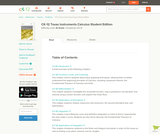Conditions of Use:
Remix and Share
Rating

CK-12's Texas Instrument Calculus Student Edition is a useful companion to a Calculus course, offering extra assignments and opportunities for students to understand course material through their graphing calculator.

Subject:
Calculus
Functions
Material Type:
Textbook
Provider:
CK-12 Foundation
Provider Set:
CK-12 FlexBook
Author:
Jordan, Lori
12/15/2010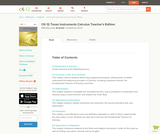Conditions of Use:
Remix and Share
Rating

CK-12's Texas Instruments Calculus Teacher's Edition is a useful companion to a Calculus course, offering extra assignments and opportunities for students to understand course material through their graphing calculator.

Subject:
Calculus
Functions
Material Type:
Textbook
Provider:
CK-12 Foundation
Provider Set:
CK-12 FlexBook
Author:
Jordan, Lori